Optimal Placement of Distributed Generation Units for Constructing Virtual Power Plant Using Binary Particle Swarm Optimization Algorithm | OMICS International
Journal of Electrical & Electronic Systems

# Optimal Placement of Distributed Generation Units for Constructing Virtual Power Plant Using Binary Particle Swarm Optimization Algorithm

Bahrami S* and Imari A

Department of Electrical Engineering, University of Isfahan, Isfahan, Iran

*Corresponding Author:
Bahrami S
Department of Electrical Engineering
University of Isfahan, Isfahan, Iran
Tel: +989372154095
E-mail: [email protected]

Received Date: February 24, 2014; Accepted Date: April 24, 2014; Published Date: May 03, 2014

Citation: Bahrami S, Imari A (2014) Optimal Placement of Distributed Generation Units for Constructing Virtual Power Plant Using Binary Particle Swarm Optimization Algorithm. J Electr Electron Syst 3:127. doi:10.4172/2332-0796.1000127

Copyright: © 2014 Bahrami S, et al. This is an open-access article distributed under the terms of the Creative Commons Attribution License, which permits unrestricted use, distribution, and reproduction in any medium, provided the original author and source are credited.

Visit for more related articles at Journal of Electrical & Electronic Systems

#### Abstract

The idea of applying distributed generation resources in distribution systems has become increasingly important due to changes in the distribution systems. Optimal sizing, location, type and installation time of DGs for constructing virtual power plant is one of the important subjects in applying distributed generation in the power system. In this paper, a new method is presented for optimal placement and type of distributed generation units and long term system planning aim to constructing virtual power plant. Minimizing the long term total cost of the system is considered as the objective function. The impacts of applying demand response on expansion planning is also investigated. The Binary particle Swarm Optimization (BPSO) method is used for solving this problem. In order to evaluate the efficiency of the proposed method, the method is tested on the IEEE 33 bus distribution system.

#### Keywords

Distributed Generation (DG); Binary particle Swarm Optimization (BPSO); Commercial Virtual Power Plant (CVPP)

#### Introduction

In recent years, the penetration of Distributed Generation (DG) units increased in power systems. Installation of DG units in distribution systems has several benefits such as reducing system losses, enhancing voltage profile, shaving peak demand, relieving overloaded distribution lines, reducing environmental impacts, increasing overall energy efficiency, and deferring investments to upgrade existing power systems . In order to receive to mentioned advantages and better system planning, optimal placement and sizing, selecting appropriate type and the installation time of DGs are necessary.

Literature review

Many studied have been implemented with the aim of power loss reduction. Genetic Algorithm (GA) , Tabu search , particle swarm optimization [4,5] and combination of PSO and GA  are some of methods which their objective function is loss reduction. In  an improved reinitialized social structures PSO algorithm has been developed for optimal placement of multiple DGs in a micro grid to minimize the real power loss within voltage and power generation limits. The analytical approach has been demonstrated in [8,9] to find the optimal size and location of DG to minimize the real power losses and enhancement in voltage profile.

In , the optimal sizing of a small isolated power system that contains renewable and/or conventional energy technologies was determined to minimize the system’s energy cost. In , DG units were placed at the most sensitive buses to improve the voltage stability. In , to determine the optimal locations of DG units in distribution system, a new multi-objective problem based on minimized power losses, enhanced reliability and improved voltage profile has been presented. Several papers have focused on the use of EAs (evolutionary algorithms), analytical methods or load flow methods to optimize the DG placement and its sizing [13,14].

The integration of DERs is another subject which affects the operation of a power system network. In this situation, distributed generation and controllable demand may have the opportunity to participate in the operation of transmission and distribution networks. However, the aggregation of many small-capacity generators into one large power generation project could improve the economics of DERs. If several DER units are linked together and are operated as one unit, the concept is often called a Virtual Power Plant (VPP). There are two types of a VPP. Commercial Virtual Power Plant (CVPP) is one type of VPP operation . The CVPP is a competitive market actor that manages the DER portfolio(s) to make optimal decisions on participation in electricity markets. From the commercial point of view, VPP as a market agent seeks to obtain the maximum benefit from the generation and the demand portfolio without considering the network constraints. Technical Virtual Power Plant (TVPP) is another type of VPP operation . The TVPP takes into consideration also the operation of the grid. The TVPP aggregates and models the response characteristics of a system containing DERs, controllable loads and networks within a single electric-geographical (grid) area. On the other hand, a TVPP consists of some DERs from the same geographic location. In this case, the impact of operation on the distribution network is also considered .

Demand Response (DR) is one of the important parts of a VPP. Demand response is established to motivate changes in electricity consumption by end users. The main benefit of DR is the improvement of power system efficiency, since a closer alignment between customers’ electricity prices and the value they place on electricity is established .

Necessity of the virtual power plant

Determining the optimal place, size, capacity, type and installing time of DGs is one of the most important subjects. If the distributed generation units are not properly installed, it may lead to disadvantages in power quality and increasing the costs of system. Virtual power plant concept is a solution for the mentioned problems.

Virtual power plant (VPP) is a flexible representation of a portfolio of DER that can be used to make contracts in the wholesale market and to offer services to the system operator. The VPP integrates the capacity of distributed energy resources and represents an operation strategy with the combination of operation parameters of each DER. Also, VPP can consider the effect of network in the output powers of integrated DGs.

Distributed generation units are the main part of virtual power plant. So, the optimal placement, type of generator, capacity and installing time of each DG unit in the system is the first stage for constructing virtual power plant. Demand response is the second element of VPP. Demand response is such a capacity which depends on the consumption patterns of consumers and means reducing the loads of consumers in response to high power market prices or emergency situations of system. Demand response programs have many advantages such as improving the reliability of the system, reducing the cost, improving the environmental problems, reducing market strength and giving better services to the consumers.

The goals of the research are including a method for determining the optimal placement, capacity and the type of DGs and the long term and short term system planning. In order to aim to the mentioned goals, the long term cost of the system must be minimized.

In this paper, a new method for optimal placement of distributed generation units for constructing virtual power plant is presented. Also, the long term planning of distribution system is performed. In order to receive to the mentioned goals, the long term total cost of system is considered as objective function and the problem is converted to an optimization problem. The Binary Particle Swarm Optimization (BPSO) method is used to solve in the proposed method.

The rest of the paper is organized as follows: Section 2 presents the problem formulation. The proposed method is described in Section 3. Section 4 describes the test system used in this paper. A brief summary of the simulation used to obtain the results, numerical results along with some observations and discussions are also included in this section. Finally, the contributions and conclusions of the paper are summarized in Section 5.

#### Problem Formulation

Optimal placement of distributed generation units in the distribution system is a very important subject for constructing virtual power plant. The problem to solve is to determine the optimal location and size of a given number of DG units. Since, the costs of the system must be optimized, in this paper, the long term total cost of system including the production cost of DGs, cost due to purchasing active power from the grid, DRs cost, upgrade cost of the system and the cost of installing smart meters in the system are considered as the objective function. The long time total cost of system is considered as following: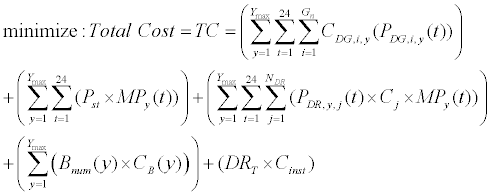(1)

The objective function has five statements. The first statement represents the production cost of GN distributed generations in all of hours for a long term period (YN). Second statement describes the cost due to purchasing energy from grid in ling term. The amount paid to customers for participation in demand response program is presented in the third part of the objective function. The fourth statement of the objective function represented the cost of system expansion in the long term. The required cost for installing smart metering devices on the customers is presented in the last statement of the objective function.

Where (CDG,I,year (PDG,I,year(t)) is the cost of ith generator in tth hour of yth year in respect of generated active power (PDG,I,year (t), iof mentioned generator. The active power received from main bus and the market price in tth hour of yth year are Ps(t) and MPyear(t), respectively. The number of lines in the network which should bundled in the yth year and the required cost for bounding one line are Bnum(Year) and CB(year), respectively. DRT is the total number of customers which installed smart meters and the cost of installing smart meters on a customer shows by Cinst. The production cost of each DG have both fixed and variable components. The fixed costs consist of capital and installation costs (C & I). It is including the cost to purchase and install a DG technology at a specified location . The variable cost includes the operation and maintenance (O & M) and the fuel cost of DG technologies (F). The capital and investment cost of generator is defined as following: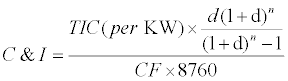(2)

Where d, n, CF and TIC are interest rate, planning period, capacity factor and total investment cost, respectively. Also, CF is defined as following .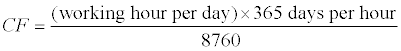(3)

So, the cost of energy (COE) produced by a DG can be considered as following: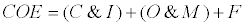(4)

Constraints

In the optimal placement and sizing of DG units for constructing VPP, the following constraints should be satisfied.

i. Unbalanced three-phase power flow equations: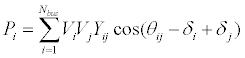(5)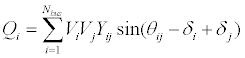(6)

ii. Active and reactive power constraints of DG units:

The output active and reactive power of each DG units must be between its minimum and maximum values: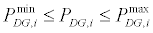(7)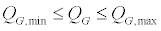(8)

iii. Active power constraints of distribution system lines: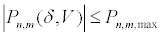(9)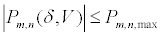(10)

iv. Voltage constraints

If the voltages along the feeder are not satisfied, optimal sizing and placement of DG are changed to the nearest values to take the feeder voltages to the voltage limits. The voltage must be kept within standard limits at each bus :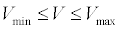(11)

#### Proposed Method

In this section, the proposed algorithm for optimal placement of DG units for constructing virtual power plant in distribution system is presented. The goals of the proposed method are determining the optimal site, capacity, and type and installation year of each DG in distribution system and long term expansion planning of distribution system. In order to aim to the mentioned goals, the proposed algorithm which shown in Figure 1 is presented. The proposed algorithm has 5 stages which are explained in following:

Stage 1: determining initial data

At the first stage of the proposed algorithm the initial data including the configuration of understudy distribution system, power market price, the operation cost of DG units and the peneteration value of demand response is determined.

Stage 2: initializing BPSO

In this stage, the number of particles (N), maximum iterations of algorithm (Nmax) and the population of PSO method are determined.

Stage 3: each particle of population is including parameters required for installing DGs in system. In this stage, DG units are placed in distribution system based on the data of each particle. Also, the production cost of each DG is determined based on installing year and using (3).

Stage 4: Optimal Power Flow (OPF)

In this stage, optimal power flow is applied to determine the optimal output actvie power of DGs, power flow of lines, voltage of buses and total cost of system. The objective function of OPF is maximization of social benefit presented in .

Stage 5: evaluating congestion in distribution system

In this stage, the condition of distribution system lines is evaluated. if there is congestion in lines, it should upgrade in expansion planning of the system.

Stage 6: determining long term system costs

Since, the long term system cost is considered as objective function, the best planning of distribution system has minimum cost in long term. So, the long term system cost is considered as fitness in BPSO method.

Stage 7: determining the best particle

Stages 1 to 6 are performed for N particle in (Nmax) iteration of BPSO method. The minimum fitness is obtained in each iteration. The best planning of the system including the site, size type and installing time of each generator has minimum cost in long term which is determined in the end of procedure shown in Figure 1.

#### Binary Particle Swarm Optimization (BPSO)

Conventional particle swarm optimization (PSO): The PSO algorithm models the behavior of a group of particles that randomly select the initial values. These particles search the problem space to find new solutions. The position and the velocity of every particle at the iteration k in the search space are described by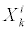and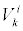, respectively. Each particle records its best local position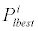. Then, the velocity of particle I in the iteration k+1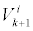is obtained from the following equation: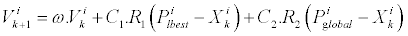(12)

Where R1 and R2 are the random functions that generate a random number between 0 and 1. Also, ω is the inertia weight factor and C1,C2 are the training coefficients. It should be noted that ω is decreased from 0.9 to 0.4 linearly. Also ω can be obtained as following: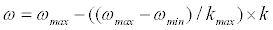(13)

Where kmax is the number of the maximum iteration. At the end of each iteration, a new position for each particle is obtained by the summation of its old position and new velocity: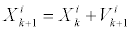(14)

Binary PSO

Binary particle swarm optimization is presented in . BPSO is used to solve discrete problems. The particle swarm formula (12) remained unchanged, except that now the position and speed of particles are integers in {0,1} and,since it is a probability, must be constrained to the interval [0,1]. A logistic transformation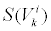is used to accomplish this modification.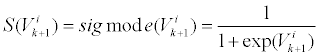(15)

The resulting change in position then is defined by the following rule: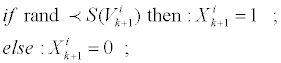(16)

Where the functionis a sigmoid limiting transformation and rand is a quasi-random number selected from a uniform distribution in [0, 1].

Application of BPSO for solving optimization problem

In the proposed method four major parameters of each DG are determined using BPSO method. These major parameters are: site, size, type and installing year of each DG. In this paper, the goal is placement of GN distributed generation in distribution system for constructing virtual power plant. Figure 2 represents the dimensions of each particle in PSO method. The number of dimension of each particle for placement GN DG unit is 4* GN. Equations (17) to (20) describe the limits of the dimensions of particles.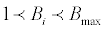(17)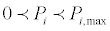(18)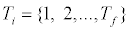(19)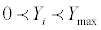(20)

Where Bmax is the maximum bus of system, Pi,max represents the maximum allowable active power of ith bus of system. It should be noted that Tf is general index for the types of DGs and ymax is the last year of the planning of the system.

#### Simulation Results

In this paper, in order to evaluate the performance of the proposed method, the method is applied on IEEE 33 bus distribution system . For this purpose, four DG is placed in distribution system by using the proposed method and an expansion planning for distribution system is performed. The results of proposed method are compared to case which no DG are placed in the system. Then, in order to evaluate the impact of demand response in distribution system, two penetration value of demand response (10% and 13%) is considered in the system and the system planning is performed.

Network information

The understudy system (IEEE 33 bus distribution system) is presented in Figure 3. The market price is in . It should be noted that the rate of annual load growth is considered as a fixed rate and equal to 5%. Also, it is supposed that all of DRs have a contract with the owner of VPP. During the contract, the amount of fines paid by customers is considered 10 percent more than the market price of electricity. All of the lines of distribution systems are considered as candidates for upgrading.

Network planning without presence of DG in the system

If in the studied system, there is no DG, all the required energy will be received from the main grid. Accordingly, long term total cost of system in 10 year period is 17.66036 million dollars. Also, the lines between buses 1-2 and 2-3 are expanded at 3rd and 10th years, respectively.

Network planning by presence of DG and absence of DR in the system

In order to evaluate the performance of the proposed method, the algorithm which is presented in Figure 1 is used to optimal placing of several DG units in the system. This case is under the conditions that there is no demand response in the system. Four DG units are placed in the 33 bus distribution system. Micro turbine and combustion turbine are considered as the types of DGs. Results of the placing are presented in Table 1. In this table, the placing, capacity, type and installing year of each DG is presented. As it is demonstrated in the Table 1, installation of one combustion turbine unit at 3rd year prohibits expansion of distribution line between 1st and 2nd buses. Therefore, the long term total cost of the system for optimal placing of four DGs in the system without presence of DR is 16.68435 million dollars.

DG number site (Bus number) Capacity (KW) Types of DG Installation time (year)
1 30 500 Micro-Turbine 5
2 18 500 Micro-Turbine 5
3 14 500 Combustion Turbine 3
4 25 500 Micro-Turbine 5

Table 1: Results of 10 years planning of the system for 4 DG placement without presence of DR.

Placement of 4 DG and network planning with 10% penetration of DR in system

For estimating the effect of demand response on 10 year planning of the system, some parts of the system loads are equipped with smart meters. Hence, in order to using DR for constructing VPP, the customers of 6th to 23rd buses (DRT=18) are equipped with telecommunicating and measuring instruments. The cost of installation of instruments on every bus (Cinst) is considered \$3000 . Penetration rate of DR in the every mentioned bus is 10%. Therefore, while the congestion occurs in the lines of the system, the owner of the VPP can cancel utmost to 10% of the loads of every buses which equipped with DR. The result of 10 year planning of system for 4 DG placement with 10% penetration of DR in the system is presented in Table 2. In this case, no transferring line needs to be expanded. Also, the long term total cost of the system is 16.18549 million dollars.

DG number site (Bus number) Capacity (KW) Types of DG Installation time (year)
1 30 500 Micro-Turbine 5
2 18 500 Micro-Turbine 5
3 14 500 Combustion Turbine 4
4 25 500 Micro-Turbine 5

Table 2: Results of 10 years planning of the system for 4 DG placement with 10% penetration of DR in the system.

Planning of the network by presence of DG and 13% penetration of DR in the system

The proposed method is applied for optimal placement of four DG 13% penetration rate of demand response in the system. The long term planning of the system and the results of the four DG placement is presented in Table 3. In this case, the total cost is 16.01971 million dollars and no distribution line needs to be expanded. It should be noted that the number of particles and iterations in BPSO method are considered 200 and 40, respectively. Figure 4 represents the convergence diagram of BPSO for the three investigated cases.

DG number site (Bus number) Capacity (KW) Types of DG Installation time (year)
1 30 500 Micro-Turbine 5
2 18 500 Micro-Turbine 5
3 14 500 Combustion Turbine 3
4 25 500 Micro-Turbine 5

Table 3: Results of 10 years planning of the system for 4 DG placement with 13% penetration of DR in the system.

#### Discussion

The cases which are studied in this paper evaluated the impact of DG and DR presence in VPP. While there is no DG in the 33 bus distribution system, the lines between 1-2and 2-3buses needs to be bundled at 3rd and 10th years, respectively. But in the case that four DG units is placed in the system, the need for expanding the lines of VPP is completely canceled. This matter shows that installing DG units in the system can lead to delay in expansion of the system. In the case presented in section 4.5, the long term total cost of the VPP is decreased at 5.53% in respect to case which no DG installed in the system. If penetration rate of DR at a part of system loads is 10%, the expansion planning of lines is delayed to 5th year. The delay is resulted by decreasing the load DRs at 1-4 years. Also, it will be completely canceled by installing one DG unit at 4th year. Also, the total cost of system in case presented in 4.5 reduced in respect to the cases NO DG-NO DR and 4 DG-NO DR 8.35% and 2/99%, respectively. In condition that rate of penetration of DR in system increases to 13%, expansion of line between 1st and 2nd buses will be delayed by load peak shaving to 5th year of planning. Finally, it will be completely canceled by installing one micro-turbine unit in 5th year at 14th bus of VPP. The total cost of the system During 10 year planning in case DG-13%DR in respect to cases NO DG-NODR, DG-NO DR, DG-10% DR is decreased to 9.29%, 3.99% and 1.02%, respectively.

#### Conclusion

In this paper a new method is presented for optimal placement of distributed generation units for constructing virtual power plant. Also, the impacts of presence of demand response have been investigated in the system and on VPP planning. Minimizing the long-term cost of VPP is considered as objective function. Hence, the production costs of distributed generation units (consist of capital and investment cost, operation and maintenance and fuel cost), the costs of expanding the lines and purchasing energy from the grid is considered. The Binary Particle Swarm Optimization (BPSO) method is used to solve the optimization problem and minimizing the objective function. The proposed method is applied for placing 4 distributed generation units in IEEE-33 bus distribution network. In order to evaluate the impact of demand response on system planning, some parts of loads of the system have been equipped with DR. Then by means of the proposed method, 10 year planning of the system has been repeated with the penetration rates of 10% and 13% penetration of DR in the system. The results demonstrate that in case of correct placing of DGs in VPP, the need for developing VPP will be delayed or removed during a specific planning and the total cost of VPP will be reduced. The cost of VPP will significantly decrease in simultaneous use of DG and DR in the system. The more penetration rate of DR in the system, the more power of owner of VPP for using of DR and as a result cost of VPP will decrease more.

#### References

Select your language of interest to view the total content in your interested language

### Article Usage

• Total views: 12840
• [From(publication date):
April-2014 - Jan 23, 2020]
• Breakdown by view type
• HTML page views : 8923Can't read the image? click here to refresh